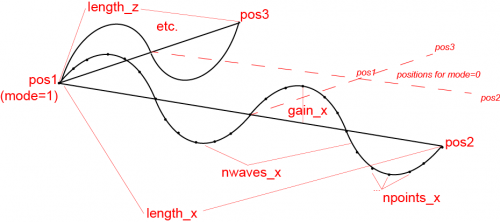# Cgo grid

Type Python Module cgo_grid.py Andreas Warnecke BSD-2-Clause This code has been put under version control in the project Pymol-script-repo

cgo_grid will generate a flowing mesh object using the points provided or the current view. By default is will generate a flowing membrane. The shape is affected substantially by the arguments!

## Usage

cgo_grid has many arguments, but not all need to be set necessarily (see arguments or examples).

 ```cgo_grid [ pos1 [, pos2 [, pos3 [, length_x [, length_z [, npoints_x [, npoints_z [, nwaves_x [, nwaves_z [, offset_x [, offset_z [, gain_x [, gain_z [, thickness [, color [, nstates [, startframe [, endframe [, mode [, view [, name [, quiet ]]]]]]]]]]]]]]]]]]]]]] ```

## Arguments

 ``` pos1 = single atom selection (='pk1') or list of 3 floats {default: [0,0,0]} pos2 = single atom selection (='pk2') or list of 3 floats {default: [1,0,0]} pos3 = single atom selection (='pk3') or list of 3 floats {default: [0,0,1]} --> the plane is defined by pos1 (origin) and vectors to pos2 and pos3, respectively length_x = : length of membrane {default: 30} length_z = : length of membrane {default: ''} # same as length_x npoints_x = : number of points(lines) along x-direction {default: ''} #will be set to give a ~1 unit grid npoints_z = : number of points(lines) along z-direction {default: ''} #will be set to give a ~1 unit grid {minimum: 1 # automatic} nwaves_x = : number of complete sin waves along object x-axis {default: 2} nwaves_z = : number of complete sin waves along object z-axis {default: ''} # same as nwaves_x define separately to adjust number of waves in each direction offset_x = phase delay (in degrees) of sin wave in x-axis can be set to affect shape and starting amplitude {default: 0} offset_z = phase delay (in degrees) of sin wave in z-axis can be set to affect shape and starting amplitude {default: ''} # same as offset_x offset_x and offset_z can be used together to phase otherwise identical objects gain_x = : multiplication factor for y-amplitude for x-direction {default: 1} gain_z = : multiplication factor for y-amplitude for z-direction {default: ''} #=gain_x thickness = : line thickness {default: 2} color = color name (e.g. 'skyblue') OR rgb-value list of 3 floats (e.g. [1.0,1.0,1.0]) OR {default: ''} // opposite of background input illegal values for random coloring nstates = : number of states; {default: 60} this setting will define how many states the object will have (per wave) and how fluent and fast the animation will be. Higher values will promote 'fluent' transitions, but decrease flow speed. Note: Frame animation cycles thought the states one at a time and needs to be set accordingly. Can also be used to phase otherwise identical objects. Set to 1 for static object {automatic minimum} startframe: specify starting frame or set (='') to use current frame set to 'append' to extend movie from the last frame {default: 1} endframe: specify end frame or set (='') to use last frame if 'append' is used for startframe, endframe becomes the number of frames to be appended instead {default: 1} Note: if start- and endframe are the same, movie animation will be skipped, the object will be loaded and can be used afterwards mode: defines positioning {default: 0}: 0: pos1 is center 1: pos1 is corner view {default: 0}: '0': off/ uses provided points to create CGO '1': overrides atom selections and uses current orienatation for positioning - pos1 = origin/center - pos2 = origin +1 in camera y - pos3 = origin +1 in camera z name: name of cgo object {default: ''} / automatic quiet: toggles output ``` Concept sketch:## Examples

The behaviour or shape of the cgo_grid object are substantially influenced by the arguments

 ```delete all set_view (\ 0.263772517, -0.113038681, 0.957937598,\ -0.040853567, 0.990910411, 0.128179103,\ -0.963716805, -0.072944686, 0.256756991,\ 0.000000000, 0.000000000, -131.816467285,\ 0.000000000, 0.000000000, 0.000000000,\ -50.008331299, 353.641235352, -20.000000000 ) #membrane cgo_grid color=blue #swimming worm, random color cgo_grid \ pos1=[0,-5,0], pos2=[1,-5,1], pos3=[0,-5,1],\ length_x=15,\ npoints_z=1,\ gain_x=2,\ gain_z=0,\ thickness=20,\ color=3,\ mode=1,\ name="worm" #Moving Ladder cgo_grid \ length_x=15,\ pos1=[0,10,0], pos2=[0,10,1], pos3=[0,9,0],\ npoints_x=2, npoints_z=30,\ name="ladder" #Roof cgo_grid \ nstates=1,\ npoints_x=15,\ npoints_z=15,\ gain_x=20,\ gain_z=20,\ nwaves_x=0.5,\ thickness=5,\ color=cyan,\ name="roof" #Boxes cgo_grid \ pos1=[0,-10,0], pos2=[1,-10,0], pos3=[0,-10,1],\ nstates=1,\ npoints_x=50,\ npoints_z=50,\ nwaves_x=0,\ color=[0.00 , 0.53 , 0.22],\ thickness=5,\ name="bottom" cgo_grid \ nstates=1,\ npoints_x=2,\ npoints_z=2,\ nwaves_x=0,\ color=gray60,\ thickness=10,\ name="top" cgo_grid \ pos1=[-15,-10,15], pos2=[-14,-10,15], pos3=[-15,-9,15],\ nstates=1,\ npoints_x=5,\ npoints_z=5,\ gain_x=0,\ gain_z=-2,\ length_z=10,\ nwaves_x=0.5,\ color=gray60,\ thickness=5,\ mode=1,\ name="front" cgo_grid \ pos1=[-15,-10,-15], pos2=[-14,-10,-15], pos3=[-15,-9,-15],\ nstates=1,\ npoints_x=5,\ npoints_z=5,\ gain_x=0,\ gain_z=2,\ length_z=10,\ nwaves_x=0.5,\ color=gray60,\ thickness=5,\ mode=1,\ name="back" set ray_trace_frames, 0 set movie_loop,1 mplay # play around with the ARGUMENTS! :-) ```